Publicité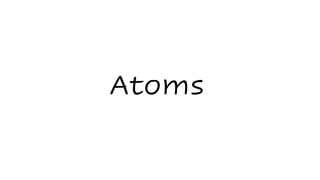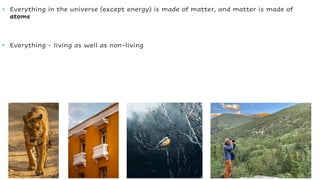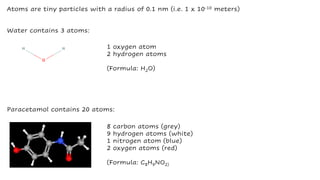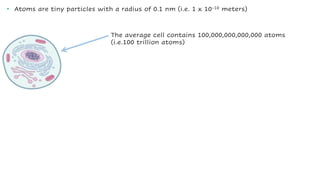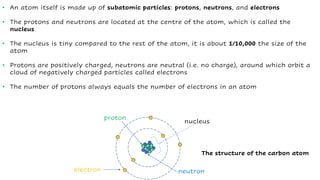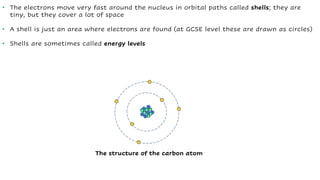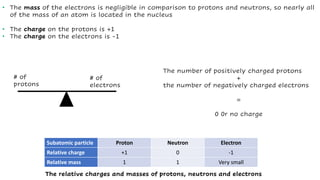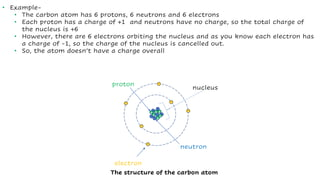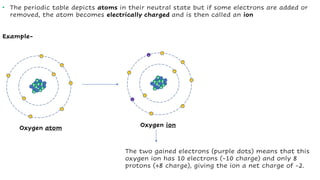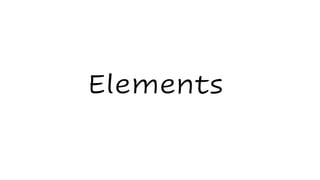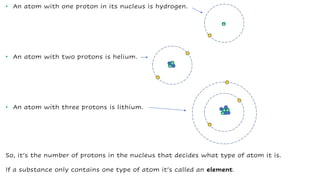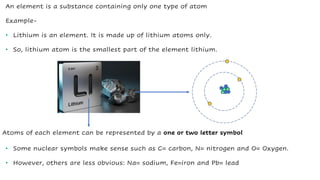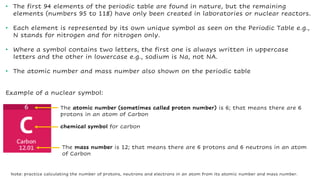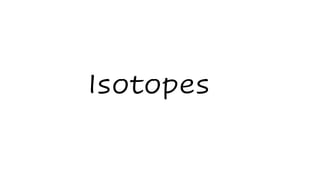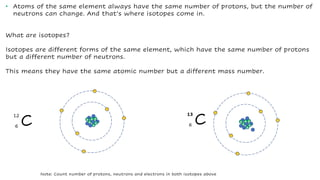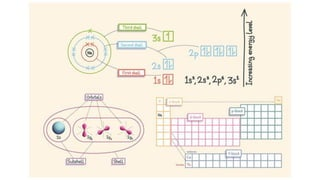1 sur 16
Publicité

### Atoms, Elements and Isotopes_GCSE Level.pdf

1. Atoms
2. • Everything in the universe (except energy) is made of matter, and matter is made of atoms • Everything - living as well as non-living
3. Paracetamol contains 20 atoms: 8 carbon atoms (grey) 9 hydrogen atoms (white) 1 nitrogen atom (blue) 2 oxygen atoms (red) (Formula: C8H9NO2) Atoms are tiny particles with a radius of 0.1 nm (i.e. 1 x 10-10 meters) 1 oxygen atom 2 hydrogen atoms (Formula: H2O) Water contains 3 atoms:
4. The average cell contains 100,000,000,000,000 atoms (i.e.100 trillion atoms) • Atoms are tiny particles with a radius of 0.1 nm (i.e. 1 x 10-10 meters)
5. • An atom itself is made up of subatomic particles: protons, neutrons, and electrons • The protons and neutrons are located at the centre of the atom, which is called the nucleus • The nucleus is tiny compared to the rest of the atom, it is about 1/10,000 the size of the atom • Protons are positively charged, neutrons are neutral (i.e. no charge), around which orbit a cloud of negatively charged particles called electrons • The number of protons always equals the number of electrons in an atom + + + + + + - - - - - - electron nucleus proton neutron The structure of the carbon atom
6. • The electrons move very fast around the nucleus in orbital paths called shells; they are tiny, but they cover a lot of space • A shell is just an area where electrons are found (at GCSE level these are drawn as circles) • Shells are sometimes called energy levels + + + + + + - - - - - - The structure of the carbon atom
7. • The mass of the electrons is negligible in comparison to protons and neutrons, so nearly all of the mass of an atom is located in the nucleus • The charge on the protons is +1 • The charge on the electrons is -1 Subatomic particle Proton Neutron Electron Relative charge +1 0 -1 Relative mass 1 1 Very small The relative charges and masses of protons, neutrons and electrons # of protons # of electrons The number of positively charged protons + the number of negatively charged electrons = 0 0r no charge
8. • Example- • The carbon atom has 6 protons, 6 neutrons and 6 electrons • Each proton has a charge of +1 and neutrons have no charge, so the total charge of the nucleus is +6 • However, there are 6 electrons orbiting the nucleus and as you know each electron has a charge of -1, so the charge of the nucleus is cancelled out. • So, the atom doesn’t have a charge overall + + + + + + - - - - - - electron nucleus proton neutron The structure of the carbon atom
9. • The periodic table depicts atoms in their neutral state but if some electrons are added or removed, the atom becomes electrically charged and is then called an ion Example- + + + + + + - - - - - - + + - - Oxygen atom + + + + + + - - - - - - + + - - Oxygen ion - - The two gained electrons (purple dots) means that this oxygen ion has 10 electrons (-10 charge) and only 8 protons (+8 charge), giving the ion a net charge of -2.
10. Elements
11. • An atom with one proton in its nucleus is hydrogen. • An atom with two protons is helium. • An atom with three protons is lithium. + + - - + - + + + - - - So, it’s the number of protons in the nucleus that decides what type of atom it is. If a substance only contains one type of atom it’s called an element.
12. An element is a substance containing only one type of atom Example- • Lithium is an element. It is made up of lithium atoms only. • So, lithium atom is the smallest part of the element lithium. + + + - - - Atoms of each element can be represented by a one or two letter symbol • Some nuclear symbols make sense such as C= carbon, N= nitrogen and O= Oxygen. • However, others are less obvious: Na= sodium, Fe=iron and Pb= lead
13. • The first 94 elements of the periodic table are found in nature, but the remaining elements (numbers 95 to 118) have only been created in laboratories or nuclear reactors. • Each element is represented by its own unique symbol as seen on the Periodic Table e.g., N stands for nitrogen and for nitrogen only. • Where a symbol contains two letters, the first one is always written in uppercase letters and the other in lowercase e.g., sodium is Na, not NA. • The atomic number and mass number also shown on the periodic table Example of a nuclear symbol: chemical symbol for carbon The atomic number (sometimes called proton number) is 6; that means there are 6 protons in an atom of Carbon The mass number is 12; that means there are 6 protons and 6 neutrons in an atom of Carbon Note: practice calculating the number of protons, neutrons and electrons in an atom from its atomic number and mass number.
14. Isotopes
15. • Atoms of the same element always have the same number of protons, but the number of neutrons can change. And that’s where isotopes come in. What are isotopes? Isotopes are different forms of the same element, which have the same number of protons but a different number of neutrons. This means they have the same atomic number but a different mass number. + + + + + + - - - - - - + + + + + + - - - - - - C 12 6 C 13 6 Note: Count number of protons, neutrons and electrons in both isotopes above
Publicité